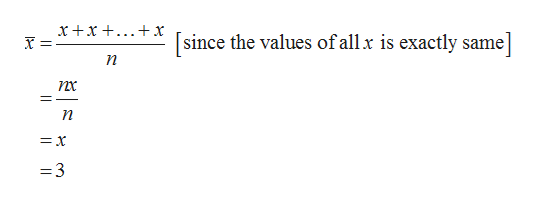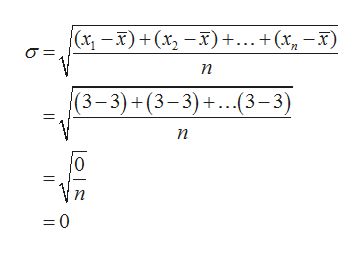Suppose that we have the data {x1, x2, ..., xn} such that x1=x2=...=xn=3. What is the mean of the data? What is the standard deviation?In the formula for the mean of the grouped data, what do you get when you have all group frequencies equal to 1?Two data sets of the same size have standard deviations s1 and s2. If s1<s2, then how do these data sets compare?

Question
1. Suppose that we have the data {x1, x2, ..., xn} such that x1=x2=...=xn=3. What is the mean of the data? What is the standard deviation?
2. In the formula for the mean of the grouped data, what do you get when you have all group frequencies equal to 1?
3. Two data sets of the same size have standard deviations s1 and s2. If s1<s2, then how do these data sets compare?
Step 1

Hey, since there are multiple questions posted, we will answer first question. If you want any specific question to be answered, then please submit that question only or specify the question number in your message.

Step 2
1. Mean of the data:

Mean is the center value of the data set. The mean is calculated by the sum of all values divided by total number of observations in the data set.

Here, x1 = x2 = … = xn = 3. That is, all the data values in the data set are exactly same.

The mean of the data is 3 as calculated as follows.help_outlineImage Transcriptionclosexx x [since the values ofallx is exactly same n =3 fullscreen
Step 3

Standard deviation of the data:

The standard deviation is the measure of spread of data in data set. In other words, the difference between the data in data sets and its mean value.

Since t...help_outlineImage Transcriptionclose(Сх — х) + (х, — х) +...+(х, — х) п (3-3) +(3-3)+...(3-3) п n = 0 fullscreen

Want to see the full answer?

See Solution

Want to see this answer and more?

Our solutions are written by experts, many with advanced degrees, and available 24/7

See Solution
Tagged in

Statistics